# Multilayer Neural Network in GML

#### drandula

##### Member
Multilayer Neural Network in GML

(Old topic with GMS2.2)
This has been project of mine for past month, I have been writing scripts in GML for Multi-Layer Neural Network.
With Scripts you can create a Neural Networks, which can be trained with given training data. Learning algorithm used here is backpropagation, which tries minimize error function based on gradients.
Scripts have been commented, and I have tried to make them easy to use and implement to game. Though I don't know what would you use them for.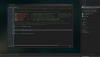Script functions support:
- Creating neural networks with any number of layers with any number of neurons
- Copy neural network in game
- Save and load neural network in external file
- Give input as array, grid, surface or sprite (currently only one color channel)
- Give learning rate. Use learning 'momentum'
- Get output as array
- Create training data, add or delete examples on the fly
- Training data accepts same inputs as neural network (useful if trying give examples of player playing)
- Set importance for training example, more important has higher learning rate
- Weight decay, Training example decay (just for fun)
- Backpropagation scripts for taking Single and Batch examples, in order or random
- Update weights and biases

I have tried how well network can learn and work with part of MNIST -dataset.
I took 10 000 images of handwritten numbers with size of 28*28, then let network learn from them, and I managed to get 97% success rate in what number my network guessed. Though this video isn't from that attempt.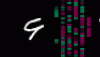(Image is input, on the right neuron should light up, topmost corresponding 9, below that 8, etc. Though video shows only 9,8)

The scripts for normal Multilayer NN are pretty much ready, but currently I am working to make Convolutional Neural Network scripts to accommodiate these other scripts.
Convolutional Neural Network has different archithecture than normal Multi-Layer Neural Network, which layers are fully connected. Cnet in other hand reduces parameters using spatial information.

I have been trying out GMS 2.3 beta for couple of days, and I have written some multi-layer perceptor code for GMS 2.3 beta. This does not work in GMS 2.2 or older version.
Currently I don't recommend using VM, as there is memory leak, which is not present using YYC compiler.

I have made only few quick short tests that it works, but haven't run any larger tests. It isn't prettiest one, but here is code for GMS 2.3 beta:

GML:
``````/// @desc    MULTI-LAYER PERCEPTOR in GML By Tero Hannula 27. April 2020
/// @desc    Multi-layer Perceptor, uses Squared Error as cost function. Tanh as activation function. Backpropagation as learning algorithm

#region /// MULTI-LAYER PERCEPTOR

function Perceptor() constructor {
/// @func    Perceptor( layer_0, layer_1, ...);
/// @desc    Creates neural network: Multi-Layer Perceptor. Add layers by stating size of them in arguments
/// @desc    Think first layer as INPUT layer, last layer as OUTPUT layer.

#region // INITIALIZE LAYERS
// Create neurons
// i: layer, j: neuron, k: next_layer_neuron.
var i_count = argument_count;

for(var i = 0; i < i_count; i++) {
var j_count = argument[i];

for(var j = 0; j < j_count; j++) {
activity[i][j] = 0;        // Activity
output[i][j] = 0;        // Output
delta[i][j] = 0;        // Delta, local error - Neuron's part in total error
bias[i][j] = random_range(-1, 1);    // Activation bias
}
}

// Create weight
var i_count = argument_count-1;

for(var i = 0; i < i_count; i++) {
var j_count = argument[i];
var k_count = argument[i+1];

for(var j = 0; j < j_count; j++) {
for(var k = 0; k < k_count; k++) {
weight[i][j][k] = random_range(-1, 1);    // Weight, kj
}
}
}

#endregion

// RESET VALUES
static Reset = function() {
/// @func Reset
var i_count = array_length(activity);

for(var i = 0; i < i_count; i++) {
var j_count = array_length(activity[i]);

for(var j = 0; j < j_count; j++) {
activity[i][j] = 0;        // Activity
output[i][j] = 0;        // Output
delta[i][j] = 0;        // Delta, local error - Neuron's part in total error
bias[i][j] = random_range(-1, 1);    // Activation bias
}
}

// Create weight
var i_count = array_length(weight);

for(var i = 0; i < i_count; i++) {
var j_count = array_length(weight[i]);

for(var j = 0; j < j_count; j++) {
var k_count = array_length(weight[i][j]);

for(var k = 0; k < k_count; k++) {
weight[i][j][k] = random_range(-1, 1);    // Weight, kj
}
}
}

}

// COST FUNCTIONS
static cost_function = function( actual_output, desired_output) {
/// @func    cost_function( actual_output, desired_output);
/// @desc    Cost function for learning algorithm, we are using Squared Error (SE)
/// @arg    {real} actual_output        What value specific output neuron is giving for result, prediction of network
/// @arg    {real}    desired_output        What value specific output neuron should have, what os actuality what we wanted. Usually compare this to training data.

return (sqr( desired_output - actual_output) / 2);    // Higher difference between prediction and actual outcome will result higher punishment
}

static cost_derivate = function( actual_output, desired_output) {
/// @func    cost_derivate( actual_output, desired_output);
/// @desc    Derivate of Cost function for learning algorithm, we are using Squared Error (SE)
/// @arg    {real} actual_output        What value specific output neuron is giving for result, prediction of network
/// @arg    {real}    desired_output        What value specific output neuron should have, what os actuality what we wanted. Usually compare this to training data.

return (actual_output - desired_output);        // Derivate of cost function - Used for backpropagation
}

// ACTIVATION FUNCTIONS
static activation_function = function( input_value) {
/// @func    activation_function( value);
/// @desc    Activation function used is TANH
/// @desc    This returns value between -1 and 1, in hard S shape.
/// @arg    {real}    value        Chosen value to squish into between -1 and 1

var value    = 2 / (1 + exp(-2 * input_value) ) - 1;    // This is TANH -activation function.
return value;
}

static activation_derivative = function( input_value) {
/// @func    activation_derivative( value);
/// @desc    Derivative for Activation function, (derivative of TANH)
/// @arg    {real}    value

var value = 1 - sqr( 2 / (1 + exp(-2 * input_value) ) - 1 );    // Derivate of tanh is "1 - sqr(tanh(x))"
return value;
}

// OUTPUT of last layer
static Output = function() {
/// @func    Output
/// @desc    Returns last layer output as array

var output_array;
var ipos = array_length(output)-1;
var j_count = array_length(output[ipos]);

for(var j = 0; j < j_count; j++) {
output_array[j] = output[ipos][j];
}

return output_array;
}

// UPDATE ACITIVTY and OUTPUTS
static Update = function( input_array) {
/// @func    Update
/// @arg    {array} input_array

// Update Input, which is first layer neurons' output
var j_count = array_length(output);

for(var j = 0; j < j_count; j++) {
output[j] = input_array[j];    // First layer neurons' output are the Input.
}

// Reset activities - default is bias
var i_count = array_length(activity);

for(var i = 1; i < i_count; i++) {
var j_count = array_length(activity[i]);

for(var j = 0; j < j_count; j++) {
activity[i][j] = bias[i][j];
}
}

// Update activity value of k-neuron
var i_count = array_length(output);

for(var i = 1; i < i_count; i++) {
var j_count = array_length(output[i]);

for(var j = 0; j < j_count; j++) {
var k_count = array_length(output[i-1]);

for(var k = 0; k < k_count; k++) {
activity[i][j] += output[i-1][k] * weight[i-1][k][j];
}
output[i][j] = activation_function(activity[i][j]);
}
}

return Output();
}

// TRAINING
static TrainSingle = function( traindata, repeat_time, learn_rate) {
/// @func    TrainSingle
/// @arg    {index}        traindata        Id of training data
/// @arg    {integer}    repeat_time
/// @arg    {real}        learn_rate

repeat( repeat_time) {
var _pos = irandom( max(0, traindata.Size()-1)); // Choose random example
Backpropagation( traindata.Input(_pos), traindata.Output(_pos));
Nudge(learn_rate, 1);
}
}

static TrainBatch = function( traindata, batch_size, repeat_time, learn_rate) {
/// @func    TrainBatch
/// @desc    Calculates mean delta from random example batch of training data
/// @arg    {index}        traindata        Id of training data
/// @arg    {integer}    batch_size        Takes average of 'random batch'.
/// @arg    {integer}    repeat_time
/// @arg    {real}        learn_rate

repeat( repeat_time) {
// Chooses random batch
repeat(batch_size) {
var _pos = irandom( max(0, traindata.Size()-1)); // Choose random example
Backpropagation( traindata.Input(_pos), traindata.Output(_pos));
}

Nudge(learn_rate, batch_size);
}
}

static TrainAll = function( traindata, repeat_time, learn_rate) {
/// @func    TrainAll
/// @desc    Calculates mean delta from all examples of training data
/// @arg    {index}        traindata        Id of training data
/// @arg    {integer}    repeat_time
/// @arg    {real}        learn_rate
var _size = traindata.Size();
repeat( repeat_time) {
for(var i = 0; i < _size; i++) {
Backpropagation( traindata.Input(i), traindata.Output(i));
}

Nudge(learn_rate, _size);
}
}

// BACKPROPAGATION - learning algorithm, calculate local errors, deltas
static Backpropagation = function( input_array, target_array) {
/// @func    Backpropagation
/// @desc    Backpropagates. Calculates cumulative delta
/// @desc    HOX! -> delta will be cumulative. Divide them by used 'example amount' to get mean delta of examples. Deltas are used to nudge weights. Nudge-function resets deltas.
/// @arg    {array}    input_array
/// @arg    {array}    output_array

// Update to get output
Update(input_array);

// Derivate activities for backpropagation
var i_count = array_length(activity)

for(var i = 0; i < i_count; i++) {
var j_count = array_length(activity[i]);

for(var j = 0; j < j_count; j++) {
activity[i][j] = activation_derivative(activity[i][j]);
}
}

// FIND ERROR ERROR in real output and target output neurons
var ipos = array_length(delta)-1;
var j_count = array_length(delta[ipos]);

for(var j = 0; j < j_count; j++) {
delta[ipos][j] = cost_derivate( output[ipos][j], target_array[j])
delta[ipos][j] *= activity[ipos][j];
}

// BACKPROPAGATE HIDDEN LAYERS - Calculate deltas
var i_count = array_length(weight)-1;

for(var i = i_count; i >= 0; i--) {
var j_count = array_length(weight[i]);

for(var j = 0; j < j_count; j++) {
var k_count = array_length(weight[i][j]);

// Calculate deltas - local errors
for(var k = 0; k < k_count; k++) {
delta[i][j] += delta[i+1][k] * weight[i][j][k];
}

delta[i][j] *= activity[i][j];
}
}
}

// NUDGE WEIGHTS AND BIASES: LEARN
static Nudge = function( learn_rate, example_count) {
/// @func    Nudge
/// @arg    {real}    learn_rate

// Update biases first, as when we update weights we reset delta
var i_count = array_length(bias)-1;

for(var i = 0; i < i_count; i++) {
var j_count = array_length(bias[i]);

for(var j = 0; j < j_count; j++) {
bias[i][j] += -learn_rate * delta[i][j] / example_count;
}
}

// Update weights
var i_count = array_length(weight);

for(var i = 0; i < i_count; i++) {
var j_count = array_length(weight[i]);

for(var j = 0; j < j_count; j++) {
var k_count = array_length(weight[i][j]);

for(var k = 0; k < k_count; k++) {
var gradient = output[i][j] * delta[i+1][k] / example_count;
weight[i][j][k] += -learn_rate * gradient; // Update weights

}
// Reset used delta
delta[i][j] = 0;
}
}

}

// DRAW NEURAL NETWORK - Something for visualizing
static Draw = function( neuron_sprite, img_index, x, y, size, scale) {
/// @func    Draw
/// @arg    {index}    neuron_sprite
/// @arg    {index}    img_index
/// @arg    {real}    x
/// @arg    {real} y
/// @arg    {real}    size
/// @arg    {real}    scale
var value, xpos, ypos, img_scale, img_color, col_scale;
var i_count = array_length(output);

for(var i = 0; i < i_count; i++) {
var j_count = array_length(output[i]);

// Draw output
for(var j = 0; j < j_count; j++) {
value = output[i][j];
xpos = x + size * (i - (i_count-1) / 2);
ypos = y + size * (j - (j_count-1) / 2);
img_scale = scale * (.75 + abs(value) * .5);
col_scale    = (abs(value) - 1) * 64;
img_color = make_color_rgb( (-value + 1)*64 + col_scale, (value + 1)*64 + col_scale, 80 + col_scale);
draw_sprite_ext( neuron_sprite, img_index, xpos, ypos, img_scale, img_scale, 0, img_color, 1);
}
}
}
}

#endregion

#region /// TRAINING DATA and EXAMPLE ADDING

function TrainingData() constructor {
/// @func    TrainingData
/// @desc    Creates base for training data, adding examples to it to be used for training Perceptor

list_input  = ds_list_create();
list_output = ds_list_create();

static AddExample = function( input_array, output_array) {
/// @arg    {array}    input_array
/// @arg    {array}    output_array

}

static ChangeExample = function( position, input_array, output_array) {
/// @func    ChangeExample
/// @arg    {integer}    position
/// @arg    {array}        input_array
/// @arg    {array}        output_array

ds_list_replace( list_input,  position, input_array);
ds_list_replace( list_output, position, output_array);
}

static Input = function(position)    {
/// @func    Input
/// @arg    {integer}    position

return (ds_list_find_value( list_input, position));
}

static Output = function(position)    {
/// @func    Output
/// @arg    {integer}    position

return (ds_list_find_value( list_output, position));
}

static Size = function() {
/// @func    Size

return (ds_list_size( list_input));
}

static DeleteExample = function( position) {
/// @func    DeleteExample
/// @arg    {integer}    position
if (position >= Size())
return;
ds_list_delete( list_input,  position);
ds_list_delete( list_output, position);
}

static Empty = function() {
/// @func    Empty

ds_list_empty( list_input);
ds_list_empty( list_output);
}

static Destroy = function() {
/// @func    Destroy

ds_list_destroy( list_input);
ds_list_destroy( list_output);
}
}

#endregion``````

Last edited:

#### var

##### Member
This is pretty cool! For a while now I've wanted to make a game that incorporates deep learning, but one of the problems is that I don't want the users to need to install tensorflow themselves. This solves that problem, though it still leaves other major problems like a single player probably wouldn't be able to generate enough training data on their own to learn anything interesting.

How much slower is it than other DL frameworks on a CPU? Is your optimizer currently just SGD? If you make a pretrained model in tensorflow or pytorch, is it feasible to convert those weights into a format that this can load?

•Cat

#### Morendral

##### Member
This looks really good, but i am a lay person. I think this would work really well if you had the resources to host a server that collected the data from each client and sent updated versions back out. That way collectively everyone would contribute to it, and the game would get harder over time

Love it!

#### drandula

##### Member
@var I don't have any coding experience outside GML and with Neural Networks I know only what I have been studying past month. So I don't know how well it would compare to real NN frameworks. But as in GMS there is no matrix calculation, and I have some 'hacky' workarounds, the speed isn't that great. This is imminient problem when you have to calculate average gradient from large batch. Getting output from given input isn't that heavy, but getting output from multiple examples, then calculating average gradient over them is heavy, and my poor computer kneels.

Lets say example: In Fullyconnected NN with input image of 64*64, there needs to be 4096 input neurons (naively), and if there is one hidden layer with 300 neurons and 10 output neurons, there will be total of 1 229 100 individual weights(!) If I want to update weights with batch of 16 examples, then I first need to calculate output of all examples to get average gradient for all these weights, and then update them. You quickly see there comes problem. This is why I want to also write scripts for Convolution neural network. This way one could reduce amount of parameters/weights, when using images as input, as it assumes spatial information is relevant.

But @var that was one great idea, if you could upload weights over from pretrained model from other framework. I need to get know how they export them to work around way to import them. If architechture is same it could be feasible.

By the way, how I store weights between layers are bit like this, ds_grid holds all weight values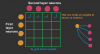Another ds_grid stores these weight-ds_grids, but also neuron-ds_grids, as neuron's contain it's activity, bias and output values. So neural network is built as nested ds_grids.

#### var

##### Member
Yeah, it'll be interesting to see what you can do once you get conv layers working! I completely skipped using basic MLPs on MNIST, so I don't know how their performance compares to a CNN.

I know that in keras it's possible to get the weights as a list of 2D arrays (technically a list of NumPy ndarrays, but it's the same thing really). They could be output in any format, e.g. JSON, which should be pretty simple to load. You'd have to manually code the same architecture in both keras and GML, but then you can load the pretrained weights straight into your ds_grids without worrying about keras' full model save format.

If you have any questions feel free to ask me! I can't promise I'll answer in a timely manner (I don't check these forums very often), but I feel like my experience might be useful. You can also message @Cat who's on here far more frequently and can bug me through other channels.

Last edited:

#### drandula

##### Member
Well I don't know how well it would do in whole MNIST, as I only did partial (about 1/6th).

Yeah, same architecture should be used for both. First same number of layers and their sizes, then same activation function. Not only weights but also biases needs to be imported. I should also think how I save my own networks in file, now I made just thing that works good enough, but isn't useful anywhere else.

I have few design choices to make for writing CNET. First do I make 3D filters support or not, as it adds complexity in structure I need to write. It also adds more arguments for user to choose from, which can also be intimidating. Though 2D filters would be just special case of 3D filters. Also it would be good to have 3D filters as RGB image colour channels are the third dimension, and with 2D filters would create different behaviour as it wouldn't know connection between channels.

For making things easier for myself I have made restrictions already, as input images and filters are rectangles, one Convolution layers filters all are same size, though can differ between convolutions layers.

I think I am gonna have problems with backpropagation for CNET, so I might ask for help sometime ^^"

I don't think these will be useful for real deep learning problems etc. as GML just can't run fast enough. But for small scale things might be, I don't know#### drandula

##### Member
Edit. Welp, real Matrix calculations would do a wonder. Anyway, currently trying to make backpropagation for 2D Cnet.

Last edited:

#### drandula

##### Member
I have been trying out beta for couple of days, and I have written some multi-layer perceptor code for GMS 2.3 beta. This does not work in GMS 2.2 or older version.
Currently I don't recommend using VM, as there is memory leak, which is not present using YYC compiler.
I am not sure how well this code works, as I wrote it from scratch. This was for learning purposes as to use GMS 2.3 beta for 'real'.
As for in GMS 2.3 scripts can have multiple functions, you need only copy this to one script and it's ready to be used.

GML:
``````/// @desc    MULTI-LAYER PERCEPTOR in GML By Tero Hannula 27. April 2020
/// @desc    Multi-layer Perceptor, uses Squared Error as cost function. Tanh as activation function. Backpropagation as learning algorithm

#region /// MULTI-LAYER PERCEPTOR

function Perceptor() constructor {
/// @func    Perceptor( layer_0, layer_1, ...);
/// @desc    Creates neural network: Multi-Layer Perceptor. Add layers by stating size of them in arguments
/// @desc    Think first layer as INPUT layer, last layer as OUTPUT layer.

#region // INITIALIZE LAYERS
// Create neurons
// i: layer, j: neuron, k: next_layer_neuron.
var i_count = argument_count;

for(var i = 0; i < i_count; i++) {
var j_count = argument[i];

for(var j = 0; j < j_count; j++) {
activity[i][j] = 0;        // Activity
output[i][j] = 0;        // Output
delta[i][j] = 0;        // Delta, local error - Neuron's part in total error
bias[i][j] = random_range(-1, 1);    // Activation bias
}
}

// Create weight
var i_count = argument_count-1;

for(var i = 0; i < i_count; i++) {
var j_count = argument[i];
var k_count = argument[i+1];

for(var j = 0; j < j_count; j++) {
for(var k = 0; k < k_count; k++) {
weight[i][j][k] = random_range(-1, 1);    // Weight, kj
}
}
}

#endregion

// RESET VALUES
static Reset = function() {
/// @func Reset
var i_count = array_length(activity);

for(var i = 0; i < i_count; i++) {
var j_count = array_length(activity[i]);

for(var j = 0; j < j_count; j++) {
activity[i][j] = 0;        // Activity
output[i][j] = 0;        // Output
delta[i][j] = 0;        // Delta, local error - Neuron's part in total error
bias[i][j] = random_range(-1, 1);    // Activation bias
}
}

// Create weight
var i_count = array_length(weight);

for(var i = 0; i < i_count; i++) {
var j_count = array_length(weight[i]);

for(var j = 0; j < j_count; j++) {
var k_count = array_length(weight[i][j]);

for(var k = 0; k < k_count; k++) {
weight[i][j][k] = random_range(-1, 1);    // Weight, kj
}
}
}

}

// COST FUNCTIONS
static cost_function = function( actual_output, desired_output) {
/// @func    cost_function( actual_output, desired_output);
/// @desc    Cost function for learning algorithm, we are using Squared Error (SE)
/// @arg    {real} actual_output        What value specific output neuron is giving for result, prediction of network
/// @arg    {real}    desired_output        What value specific output neuron should have, what os actuality what we wanted. Usually compare this to training data.

return (sqr( desired_output - actual_output) / 2);    // Higher difference between prediction and actual outcome will result higher punishment
}

static cost_derivate = function( actual_output, desired_output) {
/// @func    cost_derivate( actual_output, desired_output);
/// @desc    Derivate of Cost function for learning algorithm, we are using Squared Error (SE)
/// @arg    {real} actual_output        What value specific output neuron is giving for result, prediction of network
/// @arg    {real}    desired_output        What value specific output neuron should have, what os actuality what we wanted. Usually compare this to training data.

return (actual_output - desired_output);        // Derivate of cost function - Used for backpropagation
}

// ACTIVATION FUNCTIONS
static activation_function = function( input_value) {
/// @func    activation_function( value);
/// @desc    Activation function used is TANH
/// @desc    This returns value between -1 and 1, in hard S shape.
/// @arg    {real}    value        Chosen value to squish into between -1 and 1

var value    = 2 / (1 + exp(-2 * input_value) ) - 1;    // This is TANH -activation function.
return value;
}

static activation_derivative = function( input_value) {
/// @func    activation_derivative( value);
/// @desc    Derivative for Activation function, (derivative of TANH)
/// @arg    {real}    value

var value = 1 - sqr( 2 / (1 + exp(-2 * input_value) ) - 1 );    // Derivate of tanh is "1 - sqr(tanh(x))"
return value;
}

// OUTPUT of last layer
static Output = function() {
/// @func    Output
/// @desc    Returns last layer output as array

var output_array;
var ipos = array_length(output)-1;
var j_count = array_length(output[ipos]);

for(var j = 0; j < j_count; j++) {
output_array[j] = output[ipos][j];
}

return output_array;
}

// UPDATE ACITIVTY and OUTPUTS
static Update = function( input_array) {
/// @func    Update
/// @arg    {array} input_array

// Update Input, which is first layer neurons' output
var j_count = array_length(output);

for(var j = 0; j < j_count; j++) {
output[j] = input_array[j];    // First layer neurons' output are the Input.
}

// Reset activities - default is bias
var i_count = array_length(activity);

for(var i = 1; i < i_count; i++) {
var j_count = array_length(activity[i]);

for(var j = 0; j < j_count; j++) {
activity[i][j] = bias[i][j];
}
}

// Update activity value of k-neuron
var i_count = array_length(output);

for(var i = 1; i < i_count; i++) {
var j_count = array_length(output[i]);

for(var j = 0; j < j_count; j++) {
var k_count = array_length(output[i-1]);

for(var k = 0; k < k_count; k++) {
activity[i][j] += output[i-1][k] * weight[i-1][k][j];
}
output[i][j] = activation_function(activity[i][j]);
}
}

return Output();
}

// TRAINING
static TrainSingle = function( traindata, repeat_time, learn_rate) {
/// @func    TrainSingle
/// @arg    {index}        traindata        Id of training data
/// @arg    {integer}    repeat_time
/// @arg    {real}        learn_rate

repeat( repeat_time) {
var _pos = irandom( max(0, traindata.Size()-1)); // Choose random example
Backpropagation( traindata.Input(_pos), traindata.Output(_pos));
Nudge(learn_rate, 1);
}
}

static TrainBatch = function( traindata, batch_size, repeat_time, learn_rate) {
/// @func    TrainBatch
/// @desc    Calculates mean delta from random example batch of training data
/// @arg    {index}        traindata        Id of training data
/// @arg    {integer}    batch_size        Takes average of 'random batch'.
/// @arg    {integer}    repeat_time
/// @arg    {real}        learn_rate

repeat( repeat_time) {
// Chooses random batch
repeat(batch_size) {
var _pos = irandom( max(0, traindata.Size()-1)); // Choose random example
Backpropagation( traindata.Input(_pos), traindata.Output(_pos));
}

Nudge(learn_rate, batch_size);
}
}

static TrainAll = function( traindata, repeat_time, learn_rate) {
/// @func    TrainAll
/// @desc    Calculates mean delta from all examples of training data
/// @arg    {index}        traindata        Id of training data
/// @arg    {integer}    repeat_time
/// @arg    {real}        learn_rate
var _size = traindata.Size();
repeat( repeat_time) {
for(var i = 0; i < _size; i++) {
Backpropagation( traindata.Input(i), traindata.Output(i));
}

Nudge(learn_rate, _size);
}
}

// BACKPROPAGATION - learning algorithm, calculate local errors, deltas
static Backpropagation = function( input_array, target_array) {
/// @func    Backpropagation
/// @desc    Backpropagates. Calculates cumulative delta
/// @desc    HOX! -> delta will be cumulative. Divide them by used 'example amount' to get mean delta of examples. Deltas are used to nudge weights. Nudge-function resets deltas.
/// @arg    {array}    input_array
/// @arg    {array}    output_array

// Update to get output
Update(input_array);

// Derivate activities for backpropagation
var i_count = array_length(activity)

for(var i = 0; i < i_count; i++) {
var j_count = array_length(activity[i]);

for(var j = 0; j < j_count; j++) {
activity[i][j] = activation_derivative(activity[i][j]);
}
}

// FIND ERROR ERROR in real output and target output neurons
var ipos = array_length(delta)-1;
var j_count = array_length(delta[ipos]);

for(var j = 0; j < j_count; j++) {
delta[ipos][j] = cost_derivate( output[ipos][j], target_array[j])
delta[ipos][j] *= activity[ipos][j];
}

// BACKPROPAGATE HIDDEN LAYERS - Calculate deltas
var i_count = array_length(weight)-1;

for(var i = i_count; i >= 0; i--) {
var j_count = array_length(weight[i]);

for(var j = 0; j < j_count; j++) {
var k_count = array_length(weight[i][j]);

// Calculate deltas - local errors
for(var k = 0; k < k_count; k++) {
delta[i][j] += delta[i+1][k] * weight[i][j][k];
}

delta[i][j] *= activity[i][j];
}
}
}

// NUDGE WEIGHTS AND BIASES: LEARN
static Nudge = function( learn_rate, example_count) {
/// @func    Nudge
/// @arg    {real}    learn_rate

// Update biases first, as when we update weights we reset delta
var i_count = array_length(bias)-1;

for(var i = 0; i < i_count; i++) {
var j_count = array_length(bias[i]);

for(var j = 0; j < j_count; j++) {
bias[i][j] += -learn_rate * delta[i][j] / example_count;
}
}

// Update weights
var i_count = array_length(weight);

for(var i = 0; i < i_count; i++) {
var j_count = array_length(weight[i]);

for(var j = 0; j < j_count; j++) {
var k_count = array_length(weight[i][j]);

for(var k = 0; k < k_count; k++) {
var gradient = output[i][j] * delta[i+1][k] / example_count;
weight[i][j][k] += -learn_rate * gradient; // Update weights

}
// Reset used delta
delta[i][j] = 0;
}
}

}

// DRAW NEURAL NETWORK - Something for visualizing
static Draw = function( neuron_sprite, img_index, x, y, size, scale) {
/// @func    Draw
/// @arg    {index}    neuron_sprite
/// @arg    {index}    img_index
/// @arg    {real}    x
/// @arg    {real} y
/// @arg    {real}    size
/// @arg    {real}    scale
var value, xpos, ypos, img_scale, img_color, col_scale;
var i_count = array_length(output);

for(var i = 0; i < i_count; i++) {
var j_count = array_length(output[i]);

// Draw output
for(var j = 0; j < j_count; j++) {
value = output[i][j];
xpos = x + size * (i - (i_count-1) / 2);
ypos = y + size * (j - (j_count-1) / 2);
img_scale = scale * (.75 + abs(value) * .5);
col_scale    = (abs(value) - 1) * 64;
img_color = make_color_rgb( (-value + 1)*64 + col_scale, (value + 1)*64 + col_scale, 80 + col_scale);
draw_sprite_ext( neuron_sprite, img_index, xpos, ypos, img_scale, img_scale, 0, img_color, 1);
}
}
}
}

#endregion

#region /// TRAINING DATA and EXAMPLE ADDING

function TrainingData() constructor {
/// @func    TrainingData
/// @desc    Creates base for training data, adding examples to it to be used for training Perceptor

list_input  = ds_list_create();
list_output = ds_list_create();

static AddExample = function( input_array, output_array) {
/// @arg    {array}    input_array
/// @arg    {array}    output_array

}

static ChangeExample = function( position, input_array, output_array) {
/// @func    ChangeExample
/// @arg    {integer}    position
/// @arg    {array}        input_array
/// @arg    {array}        output_array

ds_list_replace( list_input,  position, input_array);
ds_list_replace( list_output, position, output_array);
}

static Input = function(position)    {
/// @func    Input
/// @arg    {integer}    position

return (ds_list_find_value( list_input, position));
}

static Output = function(position)    {
/// @func    Output
/// @arg    {integer}    position

return (ds_list_find_value( list_output, position));
}

static Size = function() {
/// @func    Size

return (ds_list_size( list_input));
}

static DeleteExample = function( position) {
/// @func    DeleteExample
/// @arg    {integer}    position
if (position >= Size())
return;
ds_list_delete( list_input,  position);
ds_list_delete( list_output, position);
}

static Empty = function() {
/// @func    Empty

ds_list_empty( list_input);
ds_list_empty( list_output);
}

static Destroy = function() {
/// @func    Destroy

ds_list_destroy( list_input);
ds_list_destroy( list_output);
}
}

#endregion``````

Example how to use this: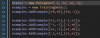And running it like: (here tanh squishes values to be between -1 and 1).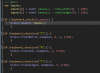And you can draw network like so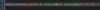In cleanup like this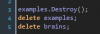Function Update updates neuron outputs by given inputs.
TrainBatch, -All or -Single trains network with given dataset with different ways:
- TrainBatch takes random batch of examples, and finds average error and updates weights accordingly
- TrainAll takes all examples of dataset, and finds average error and updates weights accordingly
- TrainSingle takes one single random example of dataset, and finds error to it and updates weights accordingly

Last edited: